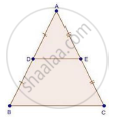Share

# In triangleAbc, D and E Are the Mid-points of Ab and Ac Respectively. Find the Ratio of the Areas of δAde and δAbc - Mathematics

Course

#### Question

In ΔABC, D and E are the mid-points of AB and AC respectively. Find the ratio of the areas of ΔADE and ΔABC

#### SolutionWe have, D and E as the mid-points of AB and AC

So, according to the mid-point theorem

DE || BC and DE =1/2BC            ...(i)

∠A = ∠A [Common]

Then, ΔADE ~ ΔABC [By AA similarity]

By area of similar triangle theorem

("Area"(triangleADE))/("Area"(triangleABC))="DE"^2/"BC"^2

=(1/2BC)^2/"BC"^2               [From (i)]

=(1/4BC^2)/"BC"^2

=1/4

Is there an error in this question or solution?

#### APPEARS IN

RD Sharma Solution for Class 10 Maths (2018 (Latest))
Chapter 7: Triangles
Ex. 7.6 | Q: 9 | Page no. 95
RD Sharma Solution for Class 10 Maths (2018 (Latest))
Chapter 7: Triangles
Ex. 7.6 | Q: 9 | Page no. 95

#### Video TutorialsVIEW ALL 

Solution In triangleAbc, D and E Are the Mid-points of Ab and Ac Respectively. Find the Ratio of the Areas of δAde and δAbc Concept: Areas of Similar Triangles.
S